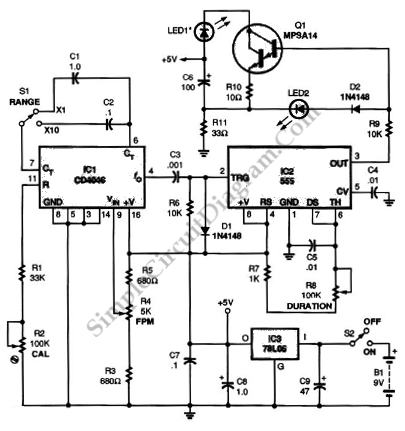# Solid-State (555 IC) Stroboscope

Stroboscope is used to measure the speed of rotation, using the effect of strobed light, where a moving object will be perceived as stationary object if the light is strobed fast enough relative to the movement speed. When applied to a rotating object, a mark on the object will be perceived as not rotating if the frequency of the strobed light is exactly equal to frequency of the stroboscope, since the light only capture the mark exactly at the precise location where the it captured in the previous cycle.

The schematic diagram shown below is a solid-state stroboscope circuit built using 555IC. This circuit is supplied by 9V battery which is kept to a more constant/stable voltage by regulating it to 5V by, and this regualtion is done by 78L05 voltage regulator when the S2 is closed (the stroboscope is powered on). The regulation is important since the stroboscope need a very stable frequency. The supply is filtered by capacitors C9 and C8. This circuit receive low-frequency square wave from the CD4046 phase-locked loop. This circuit has adjustable FPM rate from 0 to 1000. To adjust the FPM rate, Potentiometer R4-a 5000-Ω,10-turn, precision potentiometer with a built-in turns counter was used. Here is the schematic diagram of the circuit:This circuit is provided with a multiplier to extend the FPM rate range. Switch S1 is used to select that multiplier. The range of FPM rate is 0 to 1000 if the s1 is set to x1 and if S1 is set to x10 the range of FPM rate is 0- to 10,000. The capacitors C2 and C1 determine the values of the multiplier setting. The R8 is used to adjust the pulse width that produced by 555 timer. The pulse width of this stroboscope can be adjusted from 10 μs at the minimum setting to 1000μs at the maxi-mum setting.

Finally, this stroboscope uses superbright LED with a maximum rating of 100 mA for D1. This circuit also employs An MPSA14 Darlington transistor to provide an approximately 90-mA pulse. To produce that pulse, the transistor is configured as a capacitive- discharge -type current sink.